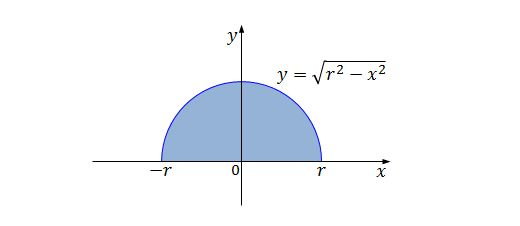Classical Mechanics

Center of a mass distribution

A bar with length $60\text{ cm}$ has linear density $\lambda=6x+12,$ where $x$ is in meters and $\lambda$ is in $\text{kg/m}.$ What is the center of mass of this bar?

A rod of length $5\text{ m}$ has density $\lambda(x)=3+x^2\text{ kg/m},$ and is positioned along the $x$-axis with left endpoint at $x=0.$ What is the center of mass of the rod?Find the center of mass of a semicircular plate of radius $4$ with uniform density.

Find the center of mass of the region bounded by the line $y=4x$ and the parabola $y=4x^2.$

What is the center of mass of the region bounded by the curves $\begin{array}{c}&y=\cos 7x, &y=0, &x=0, &x=\pi/14\end{array},$ given that the mass has uniform density?

×